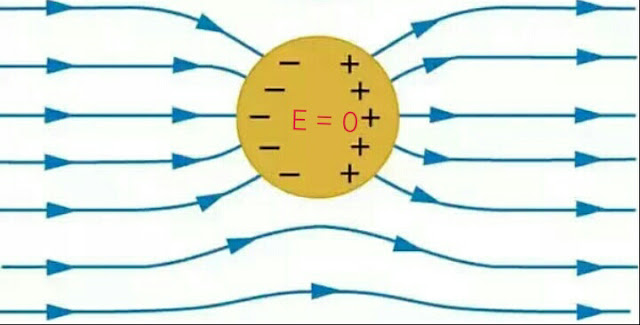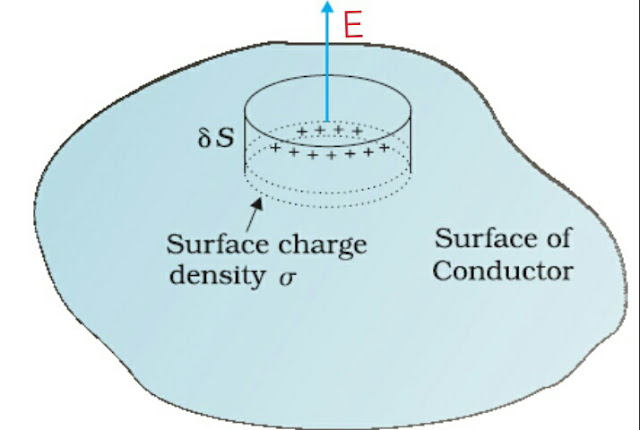# Conductors - Behaviour in an Electrostatic Field 2021.

Views

### Conductor

Three types of materials have been discovered so far and they are conductors , insulators and semiconductors. In today's article I would like to share with you an interesting information related to conductors.

Talking about conductors , they are the materials which allow large scale movement of electric charge through them. Large number of free electrons are present In conductors . These electrons are free to move throughout the whole conductor. The free electrons can be transferred out of the conductor easily with a little effort. These free electrons are also known as mobile charges means the charges that are capable of moving easily.

Talking about electrolytes, both electrons and ions are the mobile charges. Aluminium and copper are the widely used conductors. Planet Earth is also a conductor. silver is the best conductor of electricity.

### Behavior of conductors in the Electrostatic Field :

#### 1. In the interior of a conductor, net electric field intensity is zero.

we know that a conductor contains large number of free electrons. when it is placed in an electrostatic field (E₀),  each electron in it  experiences a force i.e., F = −eE₀ in a direction opposite to the direction of applied field E₀ . The importance of this force is that it causes the free electrons in the conductor to move in a direction opposite to the direction of applied electric field E₀ .Electric field inside the conductor
In figure 1, Side A of the conductor becomes positively charged due to the transfer of free electrons to the side B. Thus,  side B of the conductor becomes negatively charged. In other words,  side A of the conductor has less electrons and side B of the conductor has more electrons.

An additional field called induced electric field (EP) is created due to the redistribution of charges .

The induced electric field acts in a direction opposite to the direction of external field E₀ but its magnitude is equal to the magnitude of the external electric field inside the conductor . Hence net electric field, in the interior of the conductor placed in the electrostatic field is zero.

Points to Note:

Because of absence of electric field inside the conductor following two conditions can arise :

A) All points just under the surface of the conductor are at the same potential.

B) There will be no electric field line inside the conductor.

#### 2. Electric field just outside the charged conductor is perpendicular to the surface of the conductor.

Let us suppose that the electric field (E) is not perpendicular to the surface of the conductor. Let (E) makes an angle θ with the surface of the conductor,  then Ecosθ is tangential component of the field.

This component of the electric field called the tangential component will cause flow of charges that is surface current. But there is no surface current in electrostatics because conductor placed in an electrostatic field finally has only static charges. Thus, there is only normal component of an electric field outside the surface and no tangential component.

i. e.,                                                   Ecosθ = 0

or            cosθ = 0

or                    θ = 90       (∵ E≠ 0)

which clearly shows that the electric field just outside a charged conductor is perpendicular to the surface of the conductor at every point.

#### 3. Charge resides on the surface of a conductors.

consider a conductor which is suspended with an insulating thread. Let an excess charge q is given to the conductor. Now,  imagine a Gaussian surface as shown in the dotted line which lies just inside and near to the surface of the conductor.

We know that an electric field in the interior of the conductor is zero , so it must also be zero for all the points on the Gaussian surface which is also inside the conductor.

According to Gauss's Law, the net charge inside the Gaussian surface must be zero. If an excess charge q is not inside the Gaussian surface, then it must lie on the surface of the conductor. Thus, charge cannot reside in the interior of a conductor, it can only reside on the surface of the conductor.

#### 4. Electric field at the surface of a charged conductors.

Consider a charged conductor of irregular shape. Let  σ be the surface charge density of the conductor. Consider a Gaussian surface in the shape of a cylinder whole half portion is outside the conductor and half portion is inside the conductor.Electric field at the surface of the conductor
The Gaussian cylinder has three parts , two circular ends and one curved portion . Since , in the interior of the conductor,  electric field is zero i.e., E=0, so no electric flux is linked with one circular end which is inside the conductor of the cylinder. Also, no electric flux is linked with the curved portion of the cylinder as Electric field  E is perpendicular to this part. Electric flux passes only through the circular end ( of area vector ds) of the Gaussian cylinder which is outside the conductor.

According to Gauss's Law,

∮ E . ds   = q/ɛ₀

or          ∮ Eds cos 0⁰ = q/ɛ₀

or                    E∮ds  =  q/ɛ₀

Now                                                       ∮ds = ds     and    q = σ ds

∴          Eds = σ ds/ɛ₀

or    E  =  σ/ɛ₀

since electric field is perpendicular to the surface of the conduction , therefore , E =(σ/ɛ₀) n̂.

Points to Remember:

i) Electric charge always resides on the surface of the conductor.

ii) The magnitude of electric field intensity at the surface of a charged conductor is given by E  =  σ/ɛ₀ , where σ is the surface charge density.

iii) Net electric field intensity in the interior of a conductor placed in electrostatic field is zero.

iv) Electric field just outside the charged conductors is perpendicular to the surface of the conductor.

#### 5. Surface charge distribution may be different at different points.

Surface charge density,  σ = Electric charge /Area may be different at different points on the surface of the conductor. portions of a surface having positive charges will have positive charge density, whereas those having negative charges have negative surface charge density. A smaller surface area say conical or pointed portion of the surface of a conductor will have higher charge density.

Note:

Every conductor is an equipotential volume that is three dimensional rather than just an equipotential surface means two dimensional.

#### 6. Electric potential is constant for the entire conductor.

In general,                                        dv = - Edr

But  E = 0 for all points in the interior of a conductor. Thus from above equation, for all points in the conductor, dv = 0 or V = constant.

In other words, potential in the interior  as well as on the surface of a conductor placed in an electrostatic field is same or constant.

Conclusion:

The article has discussed about the concept of conductors as well the behavior of conductors in the Electrostatic Field. Brief equations have also been discussed in the same.

I hope the article was helpful for you. please help others through sharing on different social media platforms . I will be back with a new interesting article .Thank you for your visit.

1.The information you have shared here is really informative. I got some great knowledge from this post. Keep Posting. Custom Magnet Manufacturers in USA

2.This information is very interesting. I like your blog because the content was really impressive. Thank you for giving much of information.Magnetic coupling

3.The information you have shared here about Conductors, is really informative as it contains some great knowledge which is very helpful for me. Thanks for posting it. Keep it up. tag and test Melbourne

4.In this post, you have shared so much information about conductors - behavior in an electrostatic field. I would like to thank you for sharing such informative posts. Keep posting test & tag adelaide

5.Great tutorial you have shared here. Physics is a really difficult subject and studying such a subject online is not easy. I appreciate the author's efforts in writing these educational articles. GCSE Physics Past Papers can help students to prepare well for exams. Thank you for sharing this.